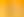How Likely is That?

# Stages1. What Does our Village Look Like?

EXPLORE

Suggested Learning Intentions

• To understand the difference between a population and a sample
• To design and conduct a survey
• To gather and evaluate categorical and numerical data and represent it using fractions, decimals, and percentages

Sample Success Criteria

• I can explain the difference between a population and a sample
• I can collect information based on questions that I pose
• I can explain the difference between numerical and categorical data
• I can use manipulatives to model the data and explain my thinking
• I can use fractions, decimals, and percentages to represent survey results
EXPLORE

2. And the Average is...

EXPLORE

Suggested Learning Intentions

• To describe, interpret and compare data using mode, median, mean and range
• To explain the difference between a population and a sample

Sample Success Criteria

• I can describe data using words such as mode, median, mean and range
• I can explain the difference between a population and a sample
• I can use a range of manipulatives to model and explain my thinking
EXPLORE

3. Four Balls

EXPLORE

Suggested Learning Intentions

• To predict the likelihood of an outcome
• To understand that some games involve random processes and that previous results have no impact upon future outcomes
• To explain the difference between dependent and independent variables

Sample Success Criteria

• I can assign probabilities to particular events
• I can use manipulatives to model events and justify my solutions
• I can use a Venn diagram to calculate probability
EXPLORE

4. Take a Chance

EXPLORE

Suggested Learning Intentions

• To understand that a ‘game of chance’ involves chance rather than skill to determine the outcome
• To list the outcomes of chance experiments
• To define and compare experimental and theoretical probability

Sample Success Criteria

• I understand that a ‘game of chance’ involves chance rather than skills to determine an outcome
• I can list the outcomes of chance experiments
• I can compare theoretical probability and experimental probability
• I can record frequencies in a tally chart and calculate the relative frequency
EXPLORE